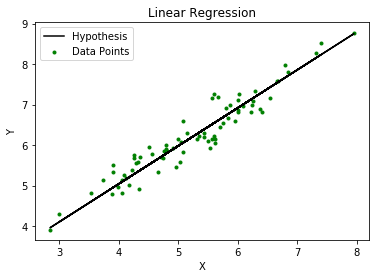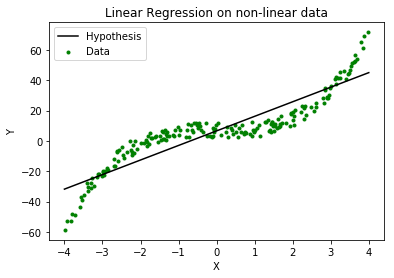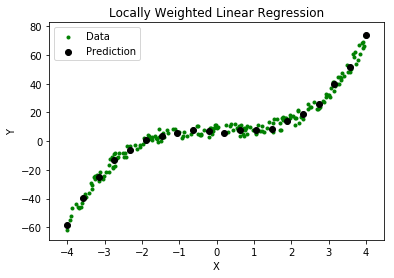Related Articles

# ML | Locally weighted Linear Regression

• Last Updated : 08 Jan, 2019

Prerequisite: ML | Linear Regression

Linear regression is a supervised learning algorithm used for computing linear relationships between input (X) and output (Y).

The steps involved in ordinary linear regression are:

Training phase: Computeto minimize the cost.Predict output: for given query point,As evident from the image below, this algorithm cannot be used for making predictions when there exists a non-linear relationship between X and Y. In such cases, locally weighted linear regression is used.### Locally Weighted Linear Regression:

Locally weighted linear regression is a non-parametric algorithm, that is, the model does not learn a fixed set of parameters as is done in ordinary linear regression. Rather parametersare computed individually for each query point. While computing, a higher “preference” is given to the points in the training set lying in the vicinity ofthan the points lying far away from.

The modified cost function is:where,is a non-negative “weight” associated with training point.
Fors lying closer to the query point, the value ofis large, while fors lying far away fromthe value ofis small.

A typical choice ofis:where,is called the bandwidth parameter and controls the rate at whichfalls with distance fromClearly, ifis smallis close to 1 and ifis largeis close to 0.

Thus, the training-set-points lying closer to the query pointcontribute more to the costthan the points lying far away from.

For example –

Consider a query point= 5.0 and letandbe two points in the training set such that= 4.9 and= 3.0.
Using the formulawith= 0.5:Thus, the weights fall exponentially as the distance betweenandincreases and so does the contribution of error in prediction forto the cost.

Consequently, while computing, we focus more on reducingfor the points lying closer to the query point (having larger value of).Steps involved in locally weighted linear regression are:

Computeto minimize the cost.Predict Output: for given query point,Points to remember:

• Locally weighted linear regression is a supervised learning algorithm.
• It a non-parametric algorithm.
• There exists No training phase. All the work is done during the testing phase/while making predictions.

Attention reader! Don’t stop learning now. Get hold of all the important Machine Learning Concepts with the Machine Learning Foundation Course at a student-friendly price and become industry ready.

My Personal Notes arrow_drop_up